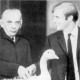# Gracious Living

Subspaces, and more continuity
November 7, 2010, 06:19
Filed under: Math, Topology | Tags: , , ,

A subspace of a topological space$X$ is a subset$Y$ of$X$ with the following topology: a set is open in$Y$ if it is of the form$U\cap Y$, with$U$ open in$X$.  We call$X$ the ambient space, and this topology the subspace or induced topology.  It’s our first way to make old topologies into new ones.  Below the fold, I discuss its implications and a couple more factoids about continuous maps.

Open sets in a subspace are open sets in the ambient space that have been “restricted” into the subspace.  So they might not always look open.  For example, take the closed unit square in$\mathbb{R}^2$ and give it the subspace topology.  Open sets in the ambient space that cross the boundary give rise to open sets of the subspace that still have some of their boundary, and thus wouldn’t be open in$\mathbb{R}^2$.  In one special circumstance, the process can, in fact, be reversed: namely, if$Y$ is open, then the open sets of$Y$ with the subspace topology are still open in$X$.

I like to give a little of the categorical perspective from time to time.  Category theorists like to define things with universal properties, which are ways of saying, using only morphisms, that a mathematical object is the best at doing whatever it does.  To begin with, observe that every subset of a set carries with it a canonical injective inclusion map of the form$i:Y\hookrightarrow X$ (the hook on the arrow denotes injectivity or inclusion), and the subspace topology on$Y$ is the coarsest topology on$Y$ making this map continuous.  (In fact,$Y$ doesn’t really need to be a subset: if we have an injective continuous map$i:Y\rightarrow X$, we can identify$Y$ with$i(Y)$ and give it a “subspace topology” this way.)  We can do even better than this though: given any map$f:Z\rightarrow Y$ for$Z$ some topological space,$f$ is continuous if and only if$i\circ f$ is continuous, where$i:Y\rightarrow X$ is the inclusion map.  Prove this, and for added points, prove that this completely defines the subspace topology (it does not hold if$Y$ has another topology).  What’s weird is how absent the topology of$X$ is from this definition.  It’s only there subtly, in the inclusion map.  I’ll leave you to ponder this further.

In general, subspaces, particularly closed subspaces, work nicely with other topological entities.  For example, it is easy to see that, if$\{B_i\}$ is a basis for$X$, then$\{B_i\cap Y\}$ is a basis for a subspace$Y$.  The subspace operation also carries over: if$Z$ is a subspace of$Y$ and$Y$ is a subspace of$X$, then$Z$ is a subspace of$X$.  Try proving this using the universal property above!  And if$X$ has two topologies,$\mathcal{O}$ and$\mathcal{O}^\prime$, with$\mathcal{O}\le\mathcal{O}^\prime$ under the coarseness ordering, then the subspace topology of$\mathcal{O}$ on some subset$Y$ of$X$ is also at least as coarse as the subspace topology of$\mathcal{O}^\prime$.

Here’s one thing that doesn’t work nicely with subspaces, and my cool example for today.  If$X$ is totally ordered, then subsets of it also acquire an induced order.  Thus, if$X$ is also a topological space, there are two natural topologies on any subset of it: the order topology, and the subspace topology.  Surprisingly, these don’t always disagree!  For example, the subspace topology of$\{0\}\cup (1,2)$ has$\{0\}$ as an open set (it is the intersection of the subspace with something like$(-1/2,1/2)$.  But under the order topology, open sets around$0$ are of the form$\{x:0\le x for some$a\in(1,2)$.  Any such set contains points of$(1,2)$, so$\{0\}$ is not open.  (As we’ll see,$\{0\}\cup(1,2)$ is even connected under the order topology.)

The situation isn’t too bad, though: define a subset$Y$ of a totally ordered set$X$ to be convex if whenever$a,b\in Y$, the whole interval$(a,b)\subset Y$.  Every convex subset of a totally ordered set has the same order topology and subspace topology.  This is easy to prove by just looking at rays, which form a basis for the order topology.

Last, some stuff about continuous maps I found in my notes.  The following functions are always continuous from$X$ to$Y$:

• A constant function sending all of$X$ to a single point of$Y$.
• If$X$ is a subspace of$Y$, the inclusion map.
• The composition of two continuous functions$f:X\rightarrow Z$ and$g:Z\rightarrow Y$.
• If we have a continuous function$f:Z\rightarrow Y$ and$X$ is a subspace of$Z$, then the restriction map$f|_X$, given by$f|_X(x)=f(x)$ for$x\in X$.
• Likewise, if$Z$ is a subspace of$Y$ and$f:X\rightarrow Z$ is continuous, we can consider$f$ to have codomain$Y$ (though it’s still the same map); this is still continuous.
• If$Y$ is a subspace of$Z$ but the range of$f:X\rightarrow Z$ is a subset of$Y$, then we can consider$f$ to have codomain$Y$, and this is still continuous.
• If$X=\bigcup_{i\in I} U_i$, where all$U_i$ are open, and we have a continuous map$f_i:U_i\rightarrow Y$ for all$i\in I$ such that $f_i=f_j$ on$U_i\cap U_j$ for all$i,j$, then we get a unique continuous map$f:X\rightarrow Y$ such that$f|_{U_i}=f_i$ for all$i\in I$.  So we can “glue” continuous maps together; in much higher math terms, continuous maps form what’s called a “sheaf.”

From now on, unless I say otherwise, you should assume any map between topological spaces to be continuous.  The exceptions will usually come when we’re proving one to be continuous, so yeah.  There’s just no reason to work with any other kind!

1 Comment so far